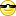Diff for "FAQ/mse" - CBU statistics Wiki
location: Diff for "FAQ/mse"
Differences between revisions 6 and 7
 ⇤ ← Revision 6 as of 2011-03-21 12:25:48 → Size: 884 Editor: PeterWatson Comment: ← Revision 7 as of 2011-03-21 12:27:15 → ⇥ Size: 908 Editor: PeterWatson Comment: Deletions are marked like this. Additions are marked like this. Line 3: Line 3: Mean square error or MSE is frequently requested by journals as a companion statistic for ANOVA and, especially, t-tests. It is the average intra-group variance. MSE is outputted in ANOVA tables but can be computed using a weighted average of the group variances. Mean square error or MSE is frequently requested by journals as a companion statistic for ANOVA and, especially, t-tests. MSE is the average intra-group variance. MSE is outputted in ANOVA tables but can be computed using a weighted average of the group variances. Line 11: Line 11: In EXCEL, for two groups (A and B ) in cells A1:A100 and B1:B100 respectively this corresponds to In EXCEL, suppose we have two groups (A and B) in cells A1:A100 and B1:B100 respectively, MSE can then be computed using

# How do I compute Mean Square Error (MSE)?

Mean square error or MSE is frequently requested by journals as a companion statistic for ANOVA and, especially, t-tests. MSE is the average intra-group variance. MSE is outputted in ANOVA tables but can be computed using a weighted average of the group variances.

Fo G groups with the i-th an SD, $$SD_text{i}$$ and sample size $$N_text{i}$$,

MSE = $$\frac{\sum_text{i} (N_text{i}-1) SD_text{i}^text{2}}{(\sum_text{i}N_text{i})-G }$$

which equals $$\frac{\sum_text{i} SD_text{i}^text{2}}{G}$$ in the special case of equal group sizes.

In EXCEL, suppose we have two groups (A andin cells A1:A100 and B1:B100 respectively, MSE can then be computed using

MSE =(SQRT(((COUNT(A1:A100)-1)+(COUNT(B1:B100)-1)))*(AVERAGE(A1:A100)-AVERAGE(B1:B100)))/(SQRT((COUNT(A1:A100)-1)*POWER(STDEV(A1:A100),2)+(COUNT(B1:B100)-1)*POWER(STDEV(B1:B100),2)))

None: FAQ/mse (last edited 2018-03-12 11:11:06 by PeterWatson)